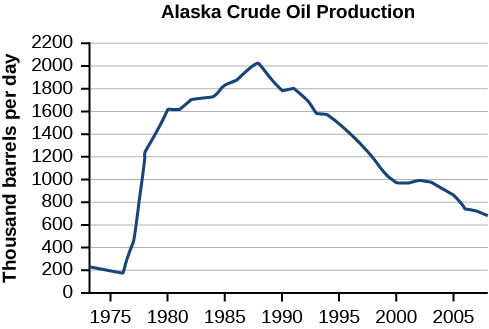# 3.2 Domain and range  (Page 4/11)

 Page 4 / 11

## Describing sets on the real-number line

Describe the intervals of values shown in [link] using inequality notation, set-builder notation, and interval notation.

To describe the values, $\text{\hspace{0.17em}}x,\text{\hspace{0.17em}}$ included in the intervals shown, we would say, “ $x\text{\hspace{0.17em}}$ is a real number greater than or equal to 1 and less than or equal to 3, or a real number greater than 5.”

 Inequality $1\le x\le 3\text{\hspace{0.17em}}\text{or}\text{\hspace{0.17em}}x>5$ Set-builder notation $\left\{x|1\le x\le 3\text{\hspace{0.17em}}\text{or}\text{\hspace{0.17em}}x>5\right\}$ Interval notation $\left[1,3\right]\cup \left(5,\infty \right)$

Remember that, when writing or reading interval notation, using a square bracket means the boundary is included in the set. Using a parenthesis means the boundary is not included in the set.

Given [link] , specify the graphed set in

1. words
2. set-builder notation
3. interval notation

1. values that are less than or equal to –2, or values that are greater than or equal to –1 and less than 3;
2. $\left\{x|x\le -2\text{\hspace{0.17em}}\text{or}\text{\hspace{0.17em}}-1\le x<3\right\}$ ;
3. $\left(-\infty ,-2\right]\cup \left[-1,3\right)$

## Finding domain and range from graphs

Another way to identify the domain and range of functions is by using graphs. Because the domain refers to the set of possible input values, the domain of a graph consists of all the input values shown on the x -axis. The range is the set of possible output values, which are shown on the y -axis. Keep in mind that if the graph continues beyond the portion of the graph we can see, the domain and range may be greater than the visible values. See [link] .

We can observe that the graph extends horizontally from $\text{\hspace{0.17em}}-5\text{\hspace{0.17em}}$ to the right without bound, so the domain is $\text{\hspace{0.17em}}\left[-5,\infty \right).\text{\hspace{0.17em}}\text{\hspace{0.17em}}$ The vertical extent of the graph is all range values $\text{\hspace{0.17em}}5\text{\hspace{0.17em}}$ and below, so the range is $\text{\hspace{0.17em}}\left(\mathrm{-\infty },5\right].\text{\hspace{0.17em}}$ Note that the domain and range are always written from smaller to larger values, or from left to right for domain, and from the bottom of the graph to the top of the graph for range.

## Finding domain and range from a graph

Find the domain and range of the function $\text{\hspace{0.17em}}f\text{\hspace{0.17em}}$ whose graph is shown in [link] .

We can observe that the horizontal extent of the graph is –3 to 1, so the domain of $\text{\hspace{0.17em}}f\text{\hspace{0.17em}}$ is $\text{\hspace{0.17em}}\left(-3,1\right].$

The vertical extent of the graph is 0 to –4, so the range is $\text{\hspace{0.17em}}\left[-4,0\right).\text{\hspace{0.17em}}$ See [link] .

## Finding domain and range from a graph of oil production

Find the domain and range of the function $\text{\hspace{0.17em}}f\text{\hspace{0.17em}}$ whose graph is shown in [link] .(credit: modification of work by the U.S. Energy Information Administration) http://www.eia.gov/dnav/pet/hist/LeafHandler.ashx?n=PET&s=MCRFPAK2&f=A.

The input quantity along the horizontal axis is “years,” which we represent with the variable $\text{\hspace{0.17em}}t\text{\hspace{0.17em}}$ for time. The output quantity is “thousands of barrels of oil per day,” which we represent with the variable $\text{\hspace{0.17em}}b\text{\hspace{0.17em}}$ for barrels. The graph may continue to the left and right beyond what is viewed, but based on the portion of the graph that is visible, we can determine the domain as $\text{\hspace{0.17em}}1973\le t\le 2008\text{\hspace{0.17em}}$ and the range as approximately $\text{\hspace{0.17em}}180\le b\le 2010.$

In interval notation, the domain is [1973, 2008], and the range is about [180, 2010]. For the domain and the range, we approximate the smallest and largest values since they do not fall exactly on the grid lines.

Given [link] , identify the domain and range using interval notation.

domain =[1950,2002] range = [47,000,000,89,000,000]

#### Questions & Answers

find to nearest one decimal place of centimeter the length of an arc of circle of radius length 12.5cm and subtending of centeral angle 1.6rad
factoring polynomial
find general solution of the Tanx=-1/root3,secx=2/root3
find general solution of the following equation
Nani
the value of 2 sin square 60 Cos 60
0.75
Lynne
0.75
Inkoom
when can I use sin, cos tan in a giving question
depending on the question
Nicholas
I am a carpenter and I have to cut and assemble a conventional roof line for a new home. The dimensions are: width 30'6" length 40'6". I want a 6 and 12 pitch. The roof is a full hip construction. Give me the L,W and height of rafters for the hip, hip jacks also the length of common jacks.
John
I want to learn the calculations
where can I get indices
I need matrices
Nasasira
hi
Raihany
Hi
Solomon
need help
Raihany
maybe provide us videos
Nasasira
Raihany
Hello
Cromwell
a
Amie
What do you mean by a
Cromwell
nothing. I accidentally press it
Amie
you guys know any app with matrices?
Khay
Ok
Cromwell
Solve the x? x=18+(24-3)=72
x-39=72 x=111
Suraj
Solve the formula for the indicated variable P=b+4a+2c, for b
Need help with this question please
b=-4ac-2c+P
Denisse
b=p-4a-2c
Suddhen
b= p - 4a - 2c
Snr
p=2(2a+C)+b
Suraj
b=p-2(2a+c)
Tapiwa
P=4a+b+2C
COLEMAN
b=P-4a-2c
COLEMAN
like Deadra, show me the step by step order of operation to alive for b
John
A laser rangefinder is locked on a comet approaching Earth. The distance g(x), in kilometers, of the comet after x days, for x in the interval 0 to 30 days, is given by g(x)=250,000csc(π30x). Graph g(x) on the interval [0, 35]. Evaluate g(5)  and interpret the information. What is the minimum distance between the comet and Earth? When does this occur? To which constant in the equation does this correspond? Find and discuss the meaning of any vertical asymptotes.
The sequence is {1,-1,1-1.....} has
circular region of radious
how can we solve this problem
Sin(A+B) = sinBcosA+cosBsinA
Prove it
Eseka
Eseka
hi
Joel
yah
immyByByByBy